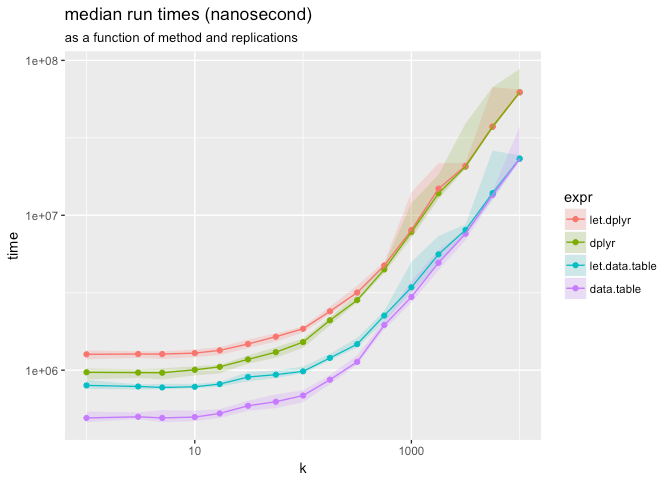# Does replyr::let work with data.table?

I’ve been asked if the adapter “`let`” from our `R` package `replyr` works with `data.table`.

My answer is: it does work. I am not a `data.table` user so I am not the one to ask if `data.table` benefits a from a non-standard evaluation to standard evaluation adapter such as `replyr::let`.

Using `replyr::let` with `data.table` looks like the following:

```library("data.table")

data("iris", package= "datasets")
iris.dt <- data.table(iris)

# non-standard evaluation, column names hard-coded
iris.dt[, mean(Sepal.Length), by=Species]

# standard evaluation, column names parameterized
let(
list(GROUPCOL='Species', DATACOL='Sepal.Length'),
iris.dt[, mean(DATACOL), by=GROUPCOL]
)

# alternate (development/Github) operator notations:

# "let in"
list(GROUPCOL='Species', DATACOL='Sepal.Length') %:%
iris.dt[, mean(DATACOL), by=GROUPCOL]

# "eval over"
iris.dt[, mean(DATACOL), by=GROUPCOL] %//%
list(GROUPCOL='Species', DATACOL='Sepal.Length')
```

I’ve generated some timings to show there is some overhead in the translation (especially on trivial examples):If any `data.table` users want to comment if this is useful or not, I’d be happy to hear from you.

Categories: Uncategorized

Tagged as:### jmount

Data Scientist and trainer at Win Vector LLC. One of the authors of Practical Data Science with R.